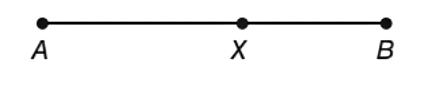Chapter 1.2, Problem 10E### Elementary Geometry for College St...

6th Edition
Daniel C. Alexander + 1 other
ISBN: 9781285195698

#### Solutions

Chapter
Section### Elementary Geometry for College St...

6th Edition
Daniel C. Alexander + 1 other
ISBN: 9781285195698
Textbook Problem
1 views

# Which symbol(s) correctly expresses the order in which the points A , B and X lie on the given line, A - X - B or A - B - X ?To determine

To find:

The symbol correctly express the order in which the point A, B and X lie on the given line, A-X-B or A-B-X.

Explanation

Calculation:

Given,

The points A and B are the endpoints of the AB¯ and the point X is in between AB¯

### Still sussing out bartleby?

Check out a sample textbook solution.

See a sample solution

#### The Solution to Your Study Problems

Bartleby provides explanations to thousands of textbook problems written by our experts, many with advanced degrees!

Get Started

#### In Exercises 2528. solve the equation by factoring. 27. x3 2x2 + 3x = 0

Applied Calculus for the Managerial, Life, and Social Sciences: A Brief Approach

#### If f(x) = cos(ln x2), find f(1).

Single Variable Calculus: Early Transcendentals, Volume I

#### Evaluate the integral. 54. (x+sinx)2dx

Single Variable Calculus: Early Transcendentals

Study Guide for Stewart's Multivariable Calculus, 8th

#### Graph: f(x)=x2+x+2x+3.

College Algebra (MindTap Course List)